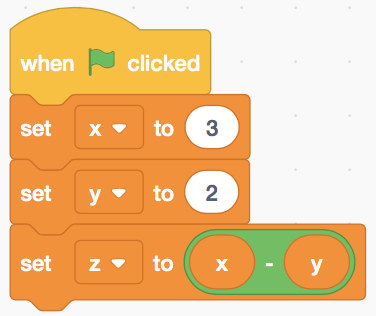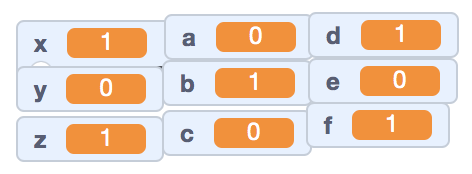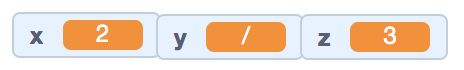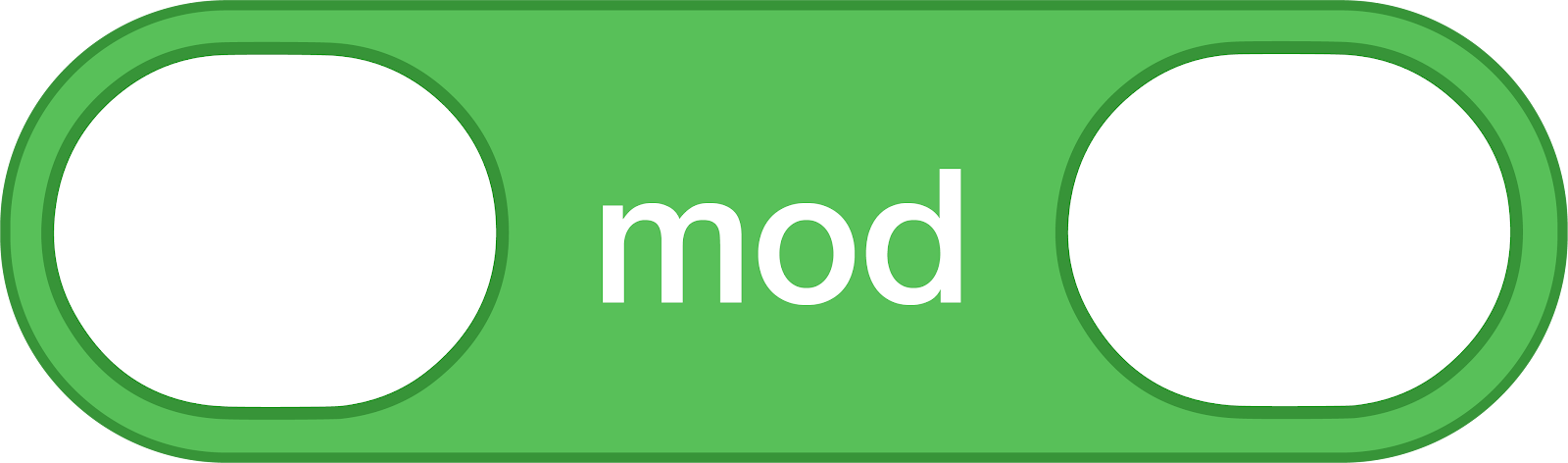### Data Processing in Scratch

Data is information. This can be just the number ‘3’ or a whole book.
Computer Programming Languages manipulate (use or change) data.
We generally split data into two categories in Scratch: numbers and strings. Strings are either single characters, words or sentences.
Examples of strings: “A”, “b”, “I like Pi”, “\$400”, “(^_^)”, “Sentences etc.”
This is a variable in Scratch. Variables contain Data.
Variables can contain either numbers or strings.

Putting Data into a Variable

Showing/Hiding Variables

Event Handler: The “When Green Flag is Clicked Block” is an event handler. This means that it executes code in response to a stimulus.

Operators: These green blocks allow you to simply manipulate data. We will first focus on number operators.
These include the traditional addition, subtraction, multiplication & division operators.

Other “Number” Operators:
We can also generate randomness and with integers b/t intervals. Scratch also has a built in “round” command.Variables + Operators: As you may have guessed, we can place variables inside of our operators. In order for the operators to work correctly, the variable needs to be holding a number.Find the final value of z in the following code:

Printing: This is a convenient way to examine the final data (output) of our project. This will “print” by having the sprite say the data on stage.Problems:

Create a 3x3 table of variables on stage (nine variables total). Set each of these variables to create a X in your table. Note: You can move variables around the screen with your mouse.Find the rounded answer for 6012.345*1232.1234 = (Write it here)

Advanced Assignment: Code a program which generates random math problems on screen with division (see the image below for an example output).Appendix 1: Advanced “Numbers” Operators
Scratch has many built-in operators that perform more advanced mathematical functions. These include the trigonometric functions (sin, cos, tan).

Scratch also contains the modulo operator which finds the remainders. Essentially, this looks at the “left-over” piece from division. For example, if I divide 4/3, it would equal 1 + 1/3. The modulo function would then return 1 because this is the piece which did not divide in evenly.